• JS实现可编辑的表格，双击可编辑，可以删除行和列增加行和列，重置，导出表格，也可以上下移动元素 源代码下载地址：http://www.zuidaima.com/share/1550463322606592.htm
JS实现可编辑的表格，双击可编辑，可以删除行和列，增加行和列，重置，导出表格，也可以上下移动元素原文：JS实现可编辑的表格，双击可编辑，可以删除行和列，增加行和列，重置，导出表格，也可以上下移动元素
源代码下载地址：http://www.zuidaima.com/share/1550463322606592.htm
源代码截图：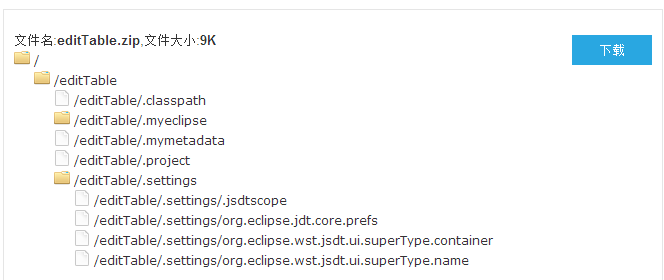展开全文js 表格 导出
• 增加行 增加 删除行 删除 function addrow(){  var c=document.getElementById('mytable');//获得表格的信息  if( c.rows.length==0){//如果是向一个空表增加一行  ...
起始状态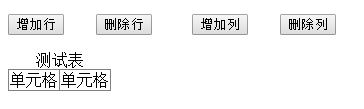增加行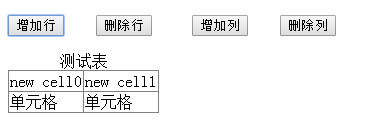增加列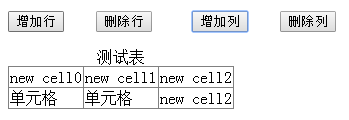删除行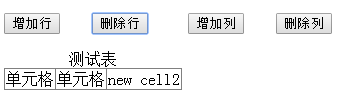删除列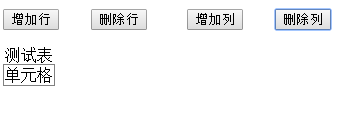<!doctype html> <html> <head> <!--动态生成指定行数和列数的可编辑表格--> <style>  </style> <script> function addrow(){   var c=document.getElementById('mytable');//获得表格的信息   if( c.rows.length==0){//如果是向一个空表增加一行  var x=c.insertRow(0);//向空表插入一行  var y=x.insertCell(0);//向新行插入一列  y.innerHTML="new cell0";   } else{ var z=c.rows.cells;//如果不是空表，首先获得表格有多少列，先获取再插入新行 var x=c.insertRow(0); for(var i=0;i<z.length;i++){//依次向新行插入表格列数的单元格      var y=x.insertCell(i); y.innerHTML="new cell"+i;  } } } function delrow(){ var x=document.getElementById("mytable"); x.deleteRow(0);//删除一行 } function addcol(){ var c=document.getElementById('mytable');//获取表格信息 var len=c.rows.length;//获得行数 var ro=c.rows.cells; var len2=ro.length;//获得列数 for(var i=0;i<len;i++){ var x=c.rows[i].insertCell(len2);//依次向每一行的末尾插入一个新列 x.innerHTML="new cell"+len2; } } function delcol(){ var c=document.getElementById('mytable');//获取表格信息 var len=c.rows.length;//获取表格的行数 var ro=c.rows.cells//获取表格的列数 var len2=ro.length-1; for(var i=0;i<len;i++){ var x=c.rows[i].deleteCell(len2);//删除每一行末尾的单元格 } } </script> <meta charset="UTF-8"> </head> <body> <p><input type="button" value="增加行" οnclick="addrow()" >&nbsp&nbsp<input type="button" value="删除行" οnclick="delrow()">&nbsp&nbsp <input type="button" value="增加列" οnclick="addcol()">&nbsp&nbsp<input type="button" value="删除列" οnclick="delcol()"></p> <table frame="border" rules="all" id="mytable"> <caption>测试表</caption> <tr> <td>单元格</td><td>单元格</td> </tr> </table> </body> </html> 
展开全文HTML
• 1、删除一、一 >>> df A B C D 0 1 3 3 4 1 5 6 7 8 2 1 1 1 1 3 2 3 2 3 #删除A，不改变原来的data数据，返回删除后的新表data_2。axis为1表示删除，0表示删除。inplace为True表示直接对原...
1、删除一行、一列
>>> df
A  B  C  D
0  1  3  3  4
1  5  6  7  8
2  1  1  1  1
3  2  3  2  3

#删除A列，不改变原来的data数据，返回删除后的新表data_2。axis为1表示删除列，0表示删除行。inplace为True表示直接对原表修改。

>>> data_2 = df.drop('A',axis=1,inplace=False)    #删除列
>>> data_2
B  C  D
0  3  3  4
1  6  7  8
2  1  1  1
3  3  2  3
>>> df.drop(0,axis=0,inplace=False)               #删除行
A  B  C  D
1  5  6  7  8
2  1  1  1  1
3  2  3  2  3
>>>

2、指定位置增加行列
>>> df
A  B  C   D
0  1  3  3   4
1  5  6  7   8
2  1  1  1   1
3  2  3  2   3
4  7  8  9  10
>>> df.insert(0,'E',[11,12,13,14,15])     #插入一列
>>> df
E  A  B  C   D
0  11  1  3  3   4
1  12  5  6  7   8
2  13  1  1  1   1
3  14  2  3  2   3
4  15  7  8  9  10
>>> df
E  A  B  C   D
0  11  1  3  3   4
1  12  5  6  7   8
2  13  1  1  1   1
3  14  2  3  2   3
4  15  7  8  9  10

df.insert(1,'调换',df.pop('A'))  #改变某一列的位置。如：先删除A列，然后在原表data中第1列插入被删掉的列。

>>> df
E  调换  B  C   D
0  11   1  3  3   4
1  12   5  6  7   8
2  13   1  1  1   1
3  14   2  3  2   3
4  15   7  8  9  10
>>> df                   字典方式添加一行，append，忽略索引
a  b  c  d
0  1  3  3  4
1  5  6  7  8
>>>
>>>
>>> row={'a':9,'b':10,'c':11,'d':12}
>>> df.append(row,ignore_index=True)
a   b   c   d
0  1   3   3   4
1  5   6   7   8
2  9  10  11  12
>>>                      用loc指定位置添加一行
>>> df.loc=[9,10,11,12]
>>> df
a   b   c   d
0  1   3   3   4
1  5   6   7   8
2  9  10  11  12
>>>

2-1、 指定位置插入一行，索引非数字
>>> df = pd.DataFrame({'A': [0, 1, 2], 'B': [3, 4, 5]},index=['c','d','f'])
>>> df
A  B
c  0  3
d  1  4
f  2  5
>>> df.loc['c']=['test','test']      ###指定位置插入一行
>>> df
A     B
c  test  test
d     1     4
f     2     5

##########如果不想替换，可以参考如下方式
pd.DataFrame(np.insert(df.values, 0, values=[10, 10], axis=0))

还有其他方式，比如把df拆分成两个df，用append，然后在合并
3、按特定顺序，插入一行，保证索引按序排列
>>> df
E  调换  B  C   D
0  11   1  3  3   4
1  12   5  6  7   8
2  13   1  1  1   1
3  14   2  3  2   3
4  15   7  8  9  10
>>>
>>> df.loc=[9,9,9,9,9]                     #插入一行，按E列的顺序，升序
>>> df
E  调换  B  C   D
0  11   1  3  3   4
1  12   5  6  7   8
2  13   1  1  1   1
3  14   2  3  2   3
4  15   7  8  9  10
5   9   9  9  9   9
>>> df.sort_values(by='E')                    #按E列排序
E  调换  B  C   D
5   9   9  9  9   9
0  11   1  3  3   4
1  12   5  6  7   8
2  13   1  1  1   1
3  14   2  3  2   3
4  15   7  8  9  10
>>> df1=df.sort_values(by='E')
>>> df1.reset_index()                        #重置索引
index   E  调换  B  C   D
0      5   9   9  9  9   9
1      0  11   1  3  3   4
2      1  12   5  6  7   8
3      2  13   1  1  1   1
4      3  14   2  3  2   3
5      4  15   7  8  9  10
>>> df2=df1.reset_index()
>>> del df2['index']
>>> df2                                      #删除掉原来的索引列index
E  调换  B  C   D
0   9   9  9  9   9
1  11   1  3  3   4
2  12   5  6  7   8
3  13   1  1  1   1
4  14   2  3  2   3
5  15   7  8  9  10
>>>                                         
参考文献：
pandas学习：对series和dataframe进行排序： https://blog.csdn.net/u014662865/article/details/59058039
Pandas set_index&reset_index ： https://blog.csdn.net/claroja/article/details/70911963
展开全文pandas dataframe
• 1、矩阵中添加： numpy.row_stack(mat, a) 2、矩阵中添加： numpy.column_stack(mat,a) #coding:utf-8 import numpy as np ...#结果是43 a=np.array([[1,2,3],[4,5,6]]) b=np.array([[1,2,3],
1、矩阵中添加行：
numpy.row_stack(mat, a)
2、矩阵中添加列：
numpy.column_stack(mat,a)

#coding:utf-8
import numpy as np
#一维向量必须reshape为规范形式
#添加行，列数必须相同
#结果是4行3列
a=np.array([[1,2,3],[4,5,6]])
b=np.array([[1,2,3],[4,5,6]])
print np.row_stack((a,b))
#添加列，行数必须相同
#[1,2,3]  [4,5]--->  [1,2,3,4,5]
a=np.array([1,2,3]).reshape(1,3)
b=np.array([4,5]).reshape(1,2)
print np.column_stack((a,b))


展开全文• //进行增加，删除表格都是对表格模型操作，与table无关 public void actionPerformed(ActionEvent e) { if (e.getSource().equals(jb1)){ tableM.addColumn("新增"); } //新增一 if (e.getSource()...
• 增加有一删除按钮，点击某的删除按钮时，删除当前   方法：  哈哈，我果然好聪明啊 1、文本框、文本框、添加按钮 2、一个DataGridView（放一个panel里），三分别是文本框、文本框、按钮     ...
• pandas.DataFrame对行和列求和及添加新行和列 pandas.DataFrame对行和列求和及添加新行和列 导入模块： from pandas import DataFrame import pandas as pd import numpy as np 生成DataFrame数据 df...
• 矩阵中添加： numpy.row_stack(mat, a) 其中a是要加入的序列 矩阵中添加： numpy.column_stack(mat,a) 其中a是要加入的序列
• 从数据库中查询数据返回datatable，简单的情况下就是不对datatable进行...可是，当问题复杂的时候，就必须对datatable进行一些操作，如：datatable的增加行、以及筛选字段组合成一张新的datatable。下面整理了一下string 数据库
• 转：...这里通过dataTable.Compute()实现计算，DataColumn.Expression实现计算。实现步骤：1、首先我们得到数据放入DataTable中，具得到方法不作说明。Code highlsql
• 使用Python的numpy的array结构，如何给矩阵增加或者一呢？ 下面提供一种方法，当然numpy还提供了很多API函数可供选择。
• 如何给 numpy 数据， 指定插入一或者一数据， insert 还是相当好玩的， 可以指定位置插入，相对于其他的还是挺强大的。 添加一数据： 方法1: 使用 insert c = np.array([[[1,2,3],[4,5,6]],[[7,8,9],...numpy
• Opencv手册： vconcat： 将多个矩阵水平的拼接起来，可用在增加某一...hconcat： 将多个矩阵垂直的拼接起来，可用在增加某一矩阵的最前行最后的。这个函数不十分紧要，一般可以用resizepushback来代替hconcat
• 最近基于公司的业务做报表的时候，很多场景都要用到排序查询和增加累加总价 1.排序查询 ----查询排序，特定300-400的数据 SELECT B.R,B.* FROM (SELECT ROWNUM R, T.* FROM SYS_ACTIVECODE T WHERE ROWNUM &...
• datagrid编辑每增加，某的单元格自动填充指定内容的两种方法datagrid
• ## Numpy中如何给矩阵增加一行或一列

万次阅读 多人点赞 2016-03-06 16:27:55
使用Python的numpy的array结构，如何给矩阵增加或者一呢？ 下面提供一种方法，当然numpy还提供了很多API函数可供选择。python numpy
• 严格来说，矩阵的长度维度是... 函数rbind()（代表row bind，按行组合）函数cbind()（代表column bind，按组合）可以给矩阵增加行。 > one  > z  > cbind( one, z )  one  [1,] 1 1 1R语言
• 在dataframe中增加或删除 首先构建一个dataframe import pandas as pd d={'one':{'a':1,'b':2,'c':3},'two':{'a':4,'b':5,'c':6},'three':{'a':7,'b':8,'c':9}} df=pd.DataFrame(d) print(df) 构建的...pandas python
• 优雅的增加，一定要优雅！df.loc['new_raw'] = '3'df Out: one two three four a 0 1 2 3 b 4 5 6 7 c 8 9 10 11 d 12 13 14 15 new_raw 3 3DataFrame
• 课程系统详细地介绍了使用R语言进行数据处理的基本思路方法。 课程能够帮助初学者快速入门数据处理。 课程通过大量的案例详细地介绍了如何使用R语言进行数据分析处理 课程操作...r语言 编程语言 云计算/大数据 大数据
• 第一种方式，直接插入一： import pandas as pd from pandas import DataFrame df3=DataFrame(np.arange(16).reshape((4,4)),index=['a','b','c','d'],columns=['one','two','three','four']) # 新插入的...pandas
• df['wine'][ran1[i]]=1 #这里难道不是对wine ran1[i]赋值为1吗， #我的结果为什么是前面全是1， 后面是-1啊 在最后一之后添加一，随机添加1/4的数据为1，剩余为-1； df.loc[len(df)] = -1 ran2=random....csv pandas python
• ## python 矩阵增加一行或一列

万次阅读 多人点赞 2017-04-05 10:47:16
矩阵增加行np.row_stack() 与 np.column_stack()“ import numpy as npa = np.array([[4, 4,], [5, 5]])c = np.row_stack((a, [8,9])) d = np.column_stack((a, [8,9])) “python numpy
•jquery
• 选择 import pandas as pd data = {'a': [0, 1], 'b': ['x', 'y'], 'c': [5, 6]} df = pd.DataFrame(data) new_df = df[['a', 'b']] print(new_df) 删除 import pandas as pd data = {'a': [0, 1], 'b': ...
• 但是显然不是我们所需要的，因为数重复了，且多了 于是，又想到第二步，可以在最外面的表中，只查到唯一的sn，所以有如下的SQL： SELECT 'SELECT id,iname,' || WMSYS.WM_CONCAT('MAX(DECODE(sn,''' || SN......